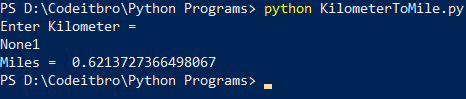# Python 3 Program to Convert Kilometres to Miles

3
489In this programming tutorial, you will learn a simple Python 3 program to convert kilometers to miles. Before moving ahead, make sure that you know about basic Python programming concepts such as variables, data types, and operators. If you love books, then do check out these best books to learn Python programming.

## Python 3 Program To Convert Kilometers to Miles

As you know, 1 KM is equal to 0.621371 Miles. Therefore, to convert kilometers to miles, you just have to divide the number entered by a user by 1.609.

Here is the Python source code you can refer:

```#Python program to convert Kilometers to Miles
KM = float(input(print("Enter Kilometer = ")))
M = KM/1.60934
print("Miles = ", M)```

## Output